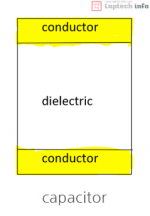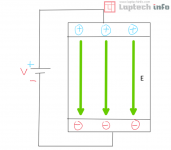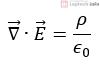What's new

# Dielectric & permittivity

### In this post, we will look at Dielectric and permittivity in a little more detail​

Dielectric is a substance that induces electric charge in Chinese characters. Dielectrics have few free charges and only bound charges. How did such a dielectric induce an electric charge, giving it the name dielectric? Let's take a look at the structure of a capacitor.A capacitor consists of two conductors and a dielectric between them, as shown above. Now let's apply voltage to the capacitor.First of all, I looked at the genome ignoring it. A constant voltage V is being applied from the outside, so both conductors will have polarity and an electric field E will be formed. Also let the distance between the two conductors be d. Pay close attention to the number of lines and the number of charges in the electric field E above. Currently there are 3 leads and 3 charges. Now consider the dielectric.As above, when an electric field is applied to the dielectric, the bound charges of the dielectric are aligned. Therefore, in the above case, one electric field line is offset by polarization. Therefore, in the above case, the offset E is the Polarization Vector P, and the directions of the two vectors are opposite to each other.

The most important things to look at are: Since a constant voltage V is applied, a constant E according to the constant voltage must be formed by the formula V=Ed. So, one more charge is needed to make up for the offset, and the charge must increase from three to four. As you can see above, the charge has changed from three to four. This is heredity. Charge is induced in the conductor by the polarization of the dielectric. Therefore, the dielectric of the dielectric means that the electric charge is induced in the conductor playing on the dielectric, rather than inducing the electric charge in the dielectric.

So what is permittivity, the higher the permittivity, the more the charge is induced. This means that a lot of charge is induced in the conductor playing in the dielectric, so for that to happen, the polarization must occur better, and in order for the polarization to occur better, there must be a lot of bound charge inside the dielectric. Therefore, if the dielectric constant is high, the amount of confined charge inside the dielectric is large, and polarization occurs well, which can be seen to induce more charge in the conductor playing in the dielectric. Therefore, the higher the permittivity, the higher the charge storage capacity. It should also not be confused that electric charge is stored in conductors rather than in dielectrics.

Therefore, finally, if we look at the dielectric, it is a material with electrical properties. Electrical properties can be said to be the degree of permittivity and susceptibility. As a final analogy, a printed circuit board (PCB) lays a conductor path on a dielectric plate, and another conductor exists under the dielectric plate. Therefore, when a voltage is applied, an electric field is formed. This electric field causes the polarization of the dielectric plate to better induce electric charges in the conductor on the dielectric plate.

So, we can compare the genome to a playground. If the playground is muddy (low dielectric constant), you won't be able to run well and you'll want to get home quickly. If the playground is a grass field (high dielectric constant), you can run around and have fun, and you will not want to go home because you want to play more. Finally, let's look at the well-known equation involving permittivity.The left-hand side of the above equation is the divergence of electric field intensity. Divergence measures the degree to which the vector field diverges from the source or enters the sink, and the right side is currently +, so it is currently diverging in a space with charge density (ρ). The magnitude of the electric field is proportional to the charge density and inversely proportional to the permittivity. As we saw above, the permittivity indicates how much bound charge is in the media. Therefore, when an electric field is applied to the media, polarization occurs and the electric field is disturbed. Therefore, the permittivity exists in the denominator as in the above equation.

I finished the content very simply, but I think the most important thing is to think about the perspective of heredity for yourself. It's something I can't understand without thinking, but I think studying will be more interesting if I understand each of those things one by one.

### Members online

No members online now.